GNSS Geodesy Innovation Interviews UAV/UAS Positioning Surveying Applications Perspective Time Navigation Mapping Policy Land Administration News Archives LBS GIS SDI Imaging E-ZineGNSS

# Simulation of Galileo E5 Signal

May 2008 | Comments Off on Simulation of Galileo E5 Signal

Fantinato Samuele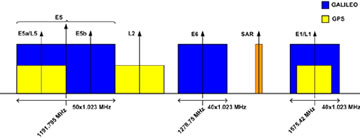Figure 1 Galileo spectrum allocation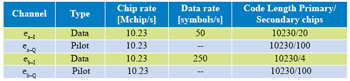Table 1 Feature of E5 components

## Simulation

The simulation is developed using Matlab. Four satellites are transmitting Galileo AltBOC(15,10) signal in steps of one millisecond.
For each transmitted signal some effects are introduced like doppler shift due to relative motion between satellite and receiver, delay, multipath channel and gaussian noise and then the overall received signal is processed with some algorithms typical of software receivers. All the blocks are simulated at baseband.

## Signal Generation

The first step in the simulation is the generation of Galileo E5 codes. In E5 signal there are four components or channels: , , and . Each component has its own code which is the product of the repetition of a primary code of 10230 chips, corresponding to 1 ms of signal, and a secondary code of different length, see Tab. 1. “Galileo Signal In Space Interface Control Document” (SIS ICD) gives the basis for generation of codes: the primary codes are generated by means of two Linear Feedback Shift Registers. For each satellite SIS ICD gives the “start values” and the values of feedback taps as polynomials in octal notation and some instructions to build correctly the LFSR. The secondary codes are only the conversion in binary representation of a hexadecimal number. Through some Matlab functions primary and secondary codes are generated for all satellites involved in simulation. As known in a Matlab simulation is necessary to define the reference time domain of each signal. The bits of the codes are then mapped in +1 BPSK values producing the discrete signals ex–y(nTc) with Tc 97.75ns that is the chip period. and are data channels with data rate reported in Tab. 1, and so the ranging codes are multiplied by two different data streams, and are pilot channels containing only the ranging codes. In conventional AltBOC the complex signal eb (t) = eb–I (t) + jeb–Q (t) is multiplied by a complex squared subcarrier er (t) = cr (t) + jsr (t) where cr (t) is the sign of a cosine function and Sr (t) is the sign of a sine function and the complex signal ea (t) = ea–I (t) + jea–Q (t) is multiplied by the complex conjugate of er (t), i.e. er *(t) obtaining the signal: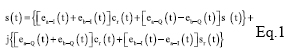In Figure 3 is represented how an AltBOC signal is built and its power spectral density in which one can recognize the two main lobes that represent the bands E5a and E5b. The Galileo AltBOC(15,10) signal structure is more complex than conventional AltBOC and has expression: where: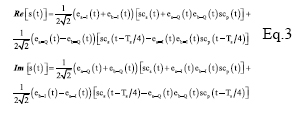where SCS(t) and SCp(t) are two subcarriers quantized in four values (Fig. 4).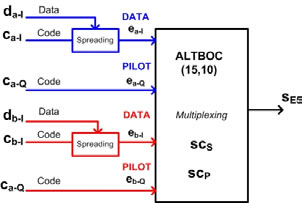Figure 2 Generation of AltBOC(15,10) signal

«Previous 1 2View All| Next»

Pages: 1 2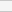(No Ratings Yet)Loading...